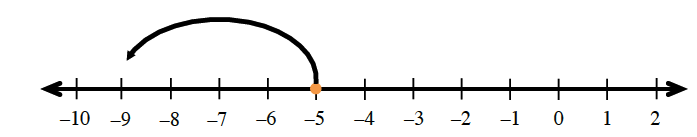### Home > MC2 > Chapter 2 > Lesson 2.1.2 > Problem2-31

2-31.

State which direction you should move on a number line from $-5$ in each expression below. Then simplify each expression.

1. $-5 + (-4)$1. $-5 - 8$

See (a).

Left, $-13$.

1. $-5 - (-6)$

$-(-6)$ means to move opposite of left $6$.
What is the opposite of left?